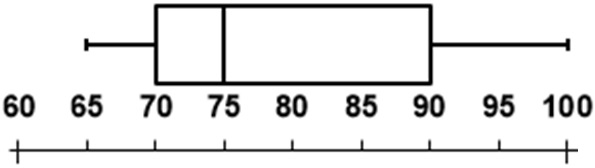© Ms. Garcia Math 7th The following box-and-whisker plot represents the scores earned on an algebra test. GR40 answersUse the box and whiser plot to answer these questions 1) What is the median score? 2) What score represents the first quartile (lower quartile)? 75 70 Answer Answer 3) What is the the minimum score? 4) What is the the maximum score? 65 100 Answer Answer 5) What score represents the third quartile (upper quartile)? 6) What is the range of the data represented in the box-and-whisker plot? 90 35 Answer Answer Name: ID: